# Addition of 2-digit, 3-digit & 4-digit Numbers

Find the sum 3473 + 53 + 682

### Solution

Step 1:

We line up the numbers vertically.Step 2:

The digits in ones column are added. 3 + 3 + 2 = 8. So 8 is written below ones column.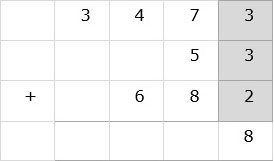Step 3:

Then the digits in tens column are added. 7 + 5 + 8 = 20. 0 is written below tens column and 2 is carried over to hundreds column.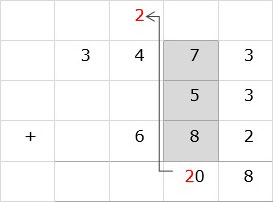Step 4:

In hundreds column, 4 + 6 + 2 = 12. 2 is written below hundreds column and 1 is carried over to thousands column.Step 5:

In thousands column, 3 + 1 = 4. 4 is written below thousands column. So the final sum is 4208.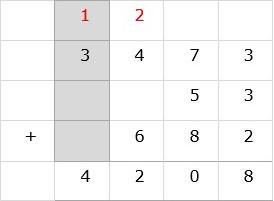Find the sum 7465 + 72 + 651

### Solution

Step 1:

We line up the numbers vertically.Step 2:

The digits in ones column are added. 5 + 2 + 1 = 8. So 8 is written below ones column.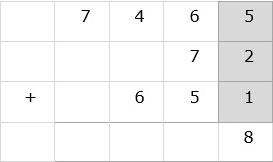Step 3:

Then the digits in tens column are added. 6 + 7 + 5 = 18. 8 is written below tens column and 1 is carried over to hundreds column.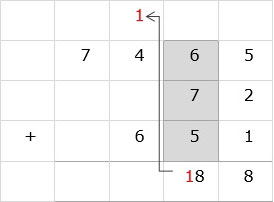Step 4:

In hundreds column, 4 + 6 + 1 = 11. 1 is written below hundreds column and 1 is carried over to thousands column.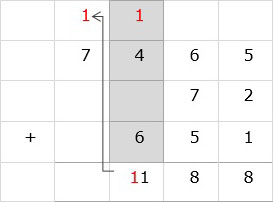Step 5:

In thousands column, 7 + 1 = 8. 8 is written below thousands column. So the final sum is 8188.